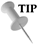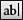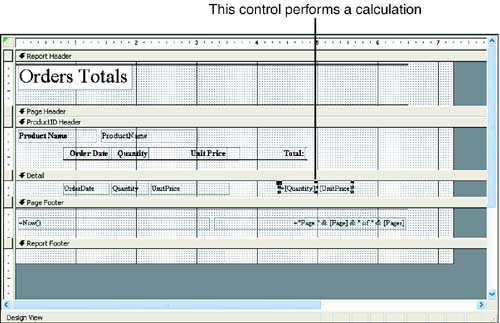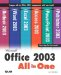# Placing a Calculation in the Report

Controls (also called text boxes in a report) most commonly display data from fields, as you've seen in the reports that you have created. However, text boxes can also hold calculations based on values in different fields.Calculations Can Be Added with the Wizard When you create a report with the Report Wizard, you are provided with options for placing summary calculations in the report. You can also add calculations to a report "after the fact" as discussed in this section.

Creating a text box holding a calculation is a bit complicated: First, you must create an unbound control/text box (that is, one that's not associated with any particular field), and then you must type the calculation into the text box. Follow these steps:

1.Click the Text Box tool in the Toolbox, and then click and drag on the report to create a text box.

2. Change the label to reflect what's going in the text box. For example, if it's sales tax or the total of your orders multiplied by the price of your various products, change the label accordingly . Position the label where you want it.

3. Click in the text box and type the formula that you want calculated. (See the following list for guidance.)

4. Click anywhere outside the text box when you finish.

Figure 18.4 shows a control that provides the total value of the orders for each item. This control multiplies the Quantity control (which is tied to a field that supplies the number of orders for each item) by the UnitPrice control (which provides the price of each item).

##### Figure 18.4. You can add controls to the report that do calculations.The formulas you enter into your calculated text box use standard mathematical symbols:

 + Add - Subtract * Multiply / Divide

All formulas begin with an equal sign (=), and all field names are in parentheses. The following are some examples:

• To calculate a total price where a control called Quantity contains the number of items and a control called Price holds the price of each item, you would multiply these data in these two controls. The formula would look like this: =[Quantity]*[UnitPrice] .

• To calculate a 25% discount off the value in the field, such as a field called Cost, you would type the formula =[Cost]*.075 .

• To add the total of the values in three fields, enter [Field1]+[Field2]+[Field3] (where Field# is the name of the field).More Room If you run out of room in the text box when typing your formula, press Shift+F2 to open a Zoom box, which gives you more room within the control.Microsoft Office 2003 All-in-One
ISBN: B005HKSHB2
EAN: N/A
Year: 2002
Pages: 660
Authors: Joe Habraken

Similar book on Amazon

flylib.com © 2008-2017.
If you may any questions please contact us: flylib@qtcs.net